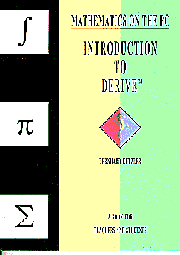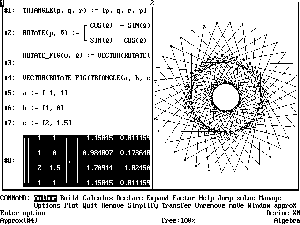﻿ Book Review: "Introduction to DERIVE" and "Mathematics with Excel" (CHEER v9 n2)## Volume 9, Issue 2, 1995## Book Reviews

• Kutzler, Bernhard, Mathematics on the PC-Introduction to DERIVE, Soft Warehouse GmbH&CoKG, ISBN 3-9500364-1-5, pp. 162, 14.50 plus VAT plus p&p, 1994.

• Sjostrand, David, Mathematics with Excel, Chartwell-Bratt Ltd, ISBN 0-86238-361-7, pp. 188, £12.95 plus VAT plus &p, 1994

Both available from Chartwell-Bratt (Publishing & Training) Ltd, Old Orchard, Bickley Road, Bromley, Kent BR1 2NE, U.K., telephone (+44) 0181-467 1956, facsimile (+44) 467 1754, Internet, philip@chartwel.demon.co.uk.

Here are two books which can be warmly recommended. Kutzler's introduction to DERIVE is a model of its kind and cannot be faulted as an introduction to DERIVE 3 use for the absolute beginner, whether at school or university. It is suitable for self-study and will not tax non-mathematical readers. Sjostrand's book can be recommended with some reservations. It attempts an important task; to show how a widely-used spreadsheet, in this case Microsoft Excel 5.0 for Windows or for Macintosh, can be used to explore mathematics at secondary school level and beyond. It would be suitable for the school or third-level student who is well supervised and has directions to a suitable mathematics text-book.

As to Kutzler, the book consists of ten short chapters together with an integrated summary and index. Each chapter ends with a command summary. Almost every page contains numerous DERIVE screen captures to illustrate algebraic and graphical examples. Format and layout are exceptionally clear. All elementary features of DERIVE use are thoroughly explored, and particular attention is paid to what can go wrong. The book does not explore DERIVE features such as the utility files, or other advanced features treated in the DERIVE user manual.

Chapter 1 introduces DERIVE as an alternative to a scientific calculator, and covers basic DERIVE commands and conventions. The next three chapters deal with polynomials and their graphs, expanding and factoring expressions and sub-expressions, and the important topic of numerical precision and its significance. How DERIVE simplifies expressions, particularly expressions involving trigonometric , exponential and logarithmic functions is next explained at length. Then vectors and matrices are introduced, followed by DERIVE's programming facility (the treatment here is very brief), before a treatment of two-and three-dimensional plots. The final chapters treat simple calculus and analytic geometry, and introduce further applications of DERIVE. As an illustration of the hundreds of examples in the book I reconstruct one only in Figure 1.#### Figure 1: A nice example from Kutzler, chapter8; one among many

This is derived from a TIF file screen capture produced by DERIVE itself. You can see a 16-colour image in the on-line version of this Review. This book is exceptionally well-written. It is an easy introduction to DERIVE and the DERIVE user manual. It prepares the reader for more advanced mathematical investigations such as those in the excellent Johnson and Evans (1992). If the price seems steep for a short paperback, it may be a relief to know that it is bundled with DERIVE Classic Student at œ29 plus VAT plus p&p for both. I cannot imagine a book of this sort being better done.

Large numbers of students at school and university have access to and use a spreadsheet without scratching the surface of the mathematical and statistical insights that such a program can provide. Berkey and Blanchard (1992) is an excellent 1300-page college calculus text with extensive materials for Pascal, Basic, Mathematica, DERIVE, and graphing scientific calculators. In a review of that text-book (Murphy (1994)) I regretted that the opportunity had not been taken to use the power of spreadsheets as a learning tool, and gave some elementary examples in numerical integration. Sj”strand attempts the important task of showing how a spreadsheet can be of use in exploring, learning and understanding mathematics. This is welcome, even if all the problems that arise in such an attempt are not entirely overcome.

Sjostrand's book has twelve chapters on various aspects of spreadsheet use. Each chapter consists of worked examples, about half a dozen in number, of mathematical problems that can be presented or solved with Excel, together with a larger number of exercises, usually not elementary, to test the reader's knowledge and develop further mathematical ideas. Very detailed step-by-step instructions, including cell references, menu commands and screen captures are provided at all stages. The spreadsheet techniques are well organised, with Visual Basic user-defined functions and macros introduced at an early stage, and continual reference back to concepts and methods already introduced. A large number of clever techniques are developed to make Excel throw light on mathematical questions. Particularly ingenious are methods for applying the laws of set theory to a specified finite set of numbers, array functions for dynamic matrix inversion, and a method of animating simple diagrams to display motion and velocity of a particle. The book is suitable for self-study, provided mathematical guidance is available from a teacher or a good text-book. A keen student will have great fun with this book and learn a lot of concrete mathematics.

There are, however, certain reservations to be made. The book was apparently written for a Swedish secondary-school audience and has slight imperfections of expressions in English; these are very minor. The organising principle is based around Excel rather than mathematical content, and the logic of spreadsheet learning and use is not the logic of mathematical learning and use. As a result, the order and development of mathematical ideas is not particularly coherent. The level of mathematics is in principle elementary, but of the sort that is in practice quite sophisticated, with examples in simple abstract algebra, set logic, analytic geometry, number theory, calculus (including integrals and differential equations), and matrices. There is also the fact that a spreadsheet has limitations as a tool for mathematical investigation, and that other software applications exist for this purpose. This is recognised by the inclusion of a chapter on using Excel to prepare input for DERIVE files, but sometimes one feels that the author is straining to show that almost any topic of school or first-year college mathematics is a natural candidate for spreadsheet treatment. Curiously, several topics in which spreadsheets still have a substantial advantage over systems like DERIVE, such as linear and non-linear programming, the mathematics of finance, and statistical estimation and inference, are not explored in detail.

To repeat: these are two good books; So”strand attempts a difficult task with tremendous enthusiasm and ingenuity; Kutzler is a model of exposition and clarity and the best starting point for any DERIVE beginner.

Barry Murphy
University of Portsmouth

References

1. Berkey, D. D. and P. Blanchard, Calculus, third edition, Saunders College Publishing, ISBN 0-03 075366-X, 1992.
2. Johnson, J. and B. Evans, Discovering Calculus with DERIVE, John Wiley and Sons, Inc., ISBN 0471-55155-4, 1992.
3. Murphy, B., "Berkey and Blanchard: a Review", Computers in Higher Education Economics Review, No. 23, pp. 40-44, November 1994.